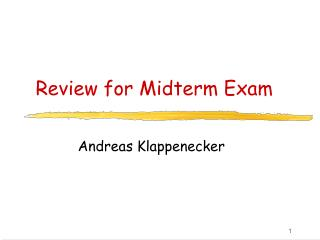# Review for Midterm Exam - PowerPoint PPT PresentationDownload PresentationReview for Midterm Exam

Review for Midterm Exam
Download Presentation## Review for Midterm Exam

- - - - - - - - - - - - - - - - - - - - - - - - - - - E N D - - - - - - - - - - - - - - - - - - - - - - - - - - -
##### Presentation Transcript

1. Review for Midterm Exam Andreas Klappenecker

2. Topics Covered • Finding Primes in the Digits of Euler's Number • Asymptotic Notations: Big Oh, Big Omega, Big Theta • Greedy Algorithms • Matroids, Matroid Embeddings • Dynamic Programming, • Amortized Analysis • Divide-and-Conquer • Disjoint Sets • Longest Common Subsequence • Graphs • Breadth First Search, Depth First Search • Topological Sorting, Strongly Connected Components • Dijkstra's Single-Source Shortest Path Algorithms • Giving Change • Bellman Ford Algorithm, Floyd-Warshall Algorithm

3. Asymptotic Notations O(g) = { f:N->R | there exists an integer n0 and a real constant C such that |f(n)| <= C|g(n)| for all n>= n0 } (g) = { f:N->R | there exists an integer n0 and a real constant c such that |f(n)| => c|g(n)| for all n>= n0 }

4. Asymptotic Notation • ½(n2+n+6) = O(n2) • 6n2 = O(n2) • 10765432n2+2n+7= (n2) • ½(n2+n+6) = (n2) • (g) = (g)  O(g) • ½(n2+n+6) = (n2)

5. Sorting Lower Bound Claim: Any comparison based sorting requires (n log n) comparisons Proof: An array a[1..n] with n elements from a totally ordered domain can be arranged in n! ways. The comparisons made by a sorting algorithm correspond to a decision tree. Each internal node contains a comparison such as a[i] < a[j]. This allows the algorithm to determine whether the elements at positions i and j are in order. Since the decision tree must be able to distinguish between n! different permutations, there are at least n! leaves in this decision tree.

6. Sorting Lower Bound A binary tree of height h does not have more than 2h leaves. Thus, we have n!< 2h Therefore, h > log n! Since log n! = log 1 + log 2 + … + log n >= n/2 log (n/2) , we can conclude that h > log n! = (n log n).

7. Divide-and-Conquer • Mergesort • Quicksort • Strassen’s matrix multiplication algorithm • Recurrence relations • Master theorem (no need to memorize)

8. Greedy Algorithms • Coin change • Huffman codes • Matroids • Kruskal’s algorithm • Matroid embeddings • Prim’s algorithm

9. Dynamic Programming • Matrix chain multiplication • Longest common subsequences

10. Modified Pseudocode for i := 1 to n do M[i,i] := 0 for d := 1 to n-1 do // diagonals for i := 1 to n-d to // rows w/ an entry on d-th diagonal j := i + d // column corresponding to row i on d-th diagonal M[i,j] := infinity for k := 1 to j-1 to M[i,j] := min(M[i,j], M[i,k]+M[k+1,j]+di-1dkdj) if previous line changed value of M[i,j] then S[i,j] := k endfor endfor endfor keep track of cheapest split point found so far: between Ak and Ak+1

11. Example M: 1: A is 30x1 2: B is 1x40 3: C is 40x10 4: D is 10x25 S: 1 1 1 2 3 3

12. Amortized Analysis • Aggregate Analysis • Accounting Method • Stacks • Counter • Disjoint Sets

13. Exam • Some short questions • Some workout problems • Lectures • Slides • Textbook • Quizzes • Homework